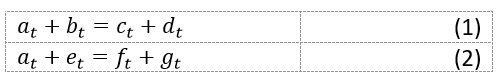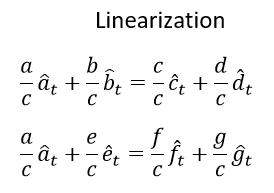# Linear approach and steady state

Hi everyone
I have a question about calibrating linear models.
Suppose we have the following two equations
(Just for simplicity; We have no parameters. and equations are for the current time and only two equations are given and the number of variables is more than the number of equations):The basic method for calibration is as followsIs it wrong to use the following approach?Is it wrong to use the second approach?
In the second approach, the two equations are separated and we no longer need to solve the system of steady state equations.
In this case, the model may be solved, but the steady state computed by Dynare may be illogical. for example c_ss=-0.04.

I am not sure I understand the question. The division by different objects is harmless unless they are 0. Similarly, the computation of ratios is innocuous. But problem can arise during loglinearizations when you suddenly take the log of something negative.

Dear professor jpfeifer
Thank you very much for your response

My question is about the need to solve the system of steady state equations in a linear method.If our method is to use a nonlinear model, we need to solve the model as a system of equations.That means we have to go through the following steps:Now if we use the linear method.
We can write linear equations as follows:In the linear method, we have to define the following items as parameters in the parameters :

a/c, b/c, c/c , d/c , e/c , f/c g/c
Of course, in dynare we write as follows:
a_c_ss, b_c_ss ,etc
Now the question is where do we get these parameters?The answer is that we get from solving the system of steady state equations.That is, we do exactly what we did in the nonlinear model here

Now if I write the linear equations as follows:That is, we normalize each equation according to one of the variables within the same equation. In this case, we no longer need to solve the Steady State equation system. That is, I do not need, for example, to get a_ss/b_ss from the first equation and then place it in the second equation and get the other parameters.I no longer need to create a precise harmony between all the equations.I just need to create a harmony between the parameters of an equation. ( between a/b b/b c/b and d/b in equation 1 and between a/e e/e f/e and g/e in equation 2 )
Is this wrong?
In the nonlinear model, we have to create this harmony between all the equations because we calculate the steady state of the variables ourselves.But in the linear model; Dynare calculates it

In large models, solving a system of steady state equations becomes very difficult. My question is, is it wrong to use the second method?